# Edraw Support Center

Get quick answers and step-by-step instructions in our support center.

## Draw Geometry

#### Draw Straight Lines

1. Select Line tool in Drawing Geometry group.
2. Position the pointer where you want the line to begin, and drag it to where you want the line to end.
3. Double-click where you want the line to begin, the Line Tool dialog will appear and specify the length and angle of the line. Then click OK.

#### Draw Arcs

1. Select Arc tool in Drawing Geometry group.
2. Position the pointer where you want the arc to begin, and drag to where you want the arc to end.
3. Double-click where you want the arc to begin, the Arc Tool dialog will appear and specify the following options. Then click OK.

X Axis Length: the width of the arc.

Y Axis Length: the height of the arc.

Type: whether the arc is open or closed.

Concave: the direction of the arc. The negative values will make a concave (inward) arc while the positive values will make a convex (outward) arc and 0 means a straight line.

#### Draw Spirals

1. Select Spiral tool.
2. Drag until the spiral is in the desired size. Drag the handle to rotate the spiral.
3. Double-click where you want the spiral to begin. In the Spiral Tool dialog, set any of the following options, and click OK.

Radius: the distance from the center to the outermost point in the spiral.

Decrease: the amount by which each wind of the spiral should decrease relative to the previous wind.

Segment Num: how many segments the spiral has and each full wind of the spiral consists of four segments.

Clockwise and Anti-Clockwise: the direction of the spiral

#### Draw Rectangles

1. Select Rectangle tool.
2. Drag and draw a rectangle or square:
• To draw a rectangle, drag diagonally until the rectangle is in the desired size.
• To draw a square, press Shift key while you drag diagonally until the square is in the desired size.
• To create a square or rectangle by specifying values, double-click where you want the center point to be. Input the width and height, and click OK.

#### Draw Rounded Rectangles

1. Select Rounded rectangle tool.
2. Drag and draw a rounded rectangle or square:
• To draw a rounded rectangle, drag diagonally until the rectangle is in the desired size.
• To draw a rounded square, press Shift key while you drag diagonally until the square is in the desired size.
• To create a rounded square or rounded rectangle by specifying values, double-click where you want the center point to be. Input a width, height and the corner radius, and then click OK.

#### Draw Ovals

1. Select Oval tool.
2. Drag and draw an oval or a circle
• To draw an oval, drag diagonally until the oval is in the desired size.
• To draw a circle, press Shift key while you drag diagonally until the circle is in the desired size.
• To create an oval by specifying values, double click where you want the center point to be. Input the width and height and then click OK.

#### Draw Polygons

1. Select Polygon tool.
2. Drag and draw a polygon until the polygon is in the desired size.
3. Double-click where you want the center of the polygon to be. Specify a radius and number of sides for the polygon, and click OK.

Note: Triangles are polygons, too! You can draw one by using the above ways.

#### Draw Stars

1. Select Star tool.
2. Double-click where you want the center of the star to be. In the Star Tool dialog, set any of the following options, and click OK.

Radius 1: the distance from the center of the star to the star’s outermost.

Radius 2: the distance from the center of the star to the star’s innermost points.

Vertex Num: how many points you want the star to have.

#### Geometry Operations

1. Select two or more shapes and the shapes must be non-grouped shapes.
2. On Symbol tab, in Geometry Operation group, choose a geometry operation option.
• Union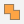: Create a shape from the perimeter of multiple overlapping shapes.
• Fragment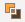: Divide multiple shapes into smaller parts based on intersecting lines or overlap.
• Combine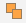: Create a shape that cuts out the overlapping portions of multiple shapes.
• Intersect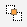: Create a shape from the area where multiple selected shapes overlap.
• Subtract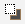: Create a shape by subtracting the overlapped area from the selected upper shape.
• Subtract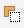: Create a shape by subtracting the overlapped area from the selected lower shape.